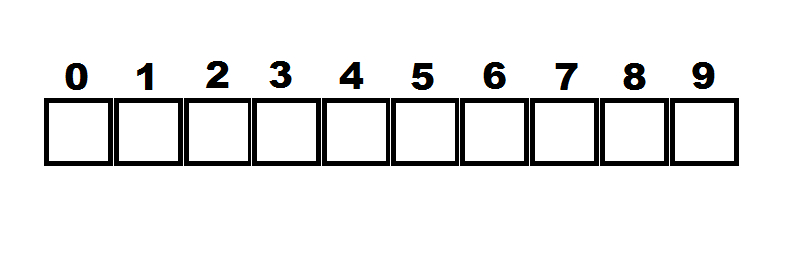# The number in the box is the number of times the number of the box occurs in the number

Logic Level 3In the figure above , there are 10 boxes numbered 1 to 9. So you have to inscribe a 10 digit number such that the digit in the first box indicates the total number of 0's in the number , the digit in the box marked "1" indicates the total number of 1's in the number, and so on to the last box, whose digit indicates the total number of 9's in the number.Find this 10 digit number.

×

Problem Loading...

Note Loading...

Set Loading...